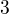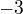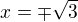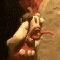# Matematické Fórum

Nevíte-li si rady s jakýmkoliv matematickým problémem, toto místo je pro vás jako dělané.

Nástěnka
22. 8. 2021 (L) Přecházíme zpět na doménu forum.matweb.cz!
04.11.2016 (Jel.) Čtete, prosím, před vložení dotazu, děkuji!
23.10.2013 (Jel.) Zkuste před zadáním dotazu použít některý z online-nástrojů, konzultovat použití můžete v sekci CAS.

Nejste přihlášen(a). Přihlásit

## #26 10. 07. 2020 07:25

vlado_bbModerátor
Příspěvky: 5867
Škola:
Reputace:   133

### Re: Systém diferenciálních rovnic

↑↑ Dacu:Of course he is wrong,$3$ and$-3$ are not roots of$x^2-3=0$.

Offline

## #27 10. 07. 2020 19:42 — Editoval Dacu (10. 07. 2020 19:46)

DacuPříspěvky: 65
Pozice: inženýr
Reputace:

### Re: Systém diferenciálních rovnic

↑↑ Dacu:Of course he is wrong,$3$ and$-3$ are not roots of$x^2-3=0$.

Hello,

I made the same mistake and you saw that I corrected it ....Bati had to write$x=\mp \sqrt{3}$....

It is wrong what Bati says, namely:
"Let$c_1=c_2=0$ Then$f(x)-3g'(x)=0$ for all$x\in\mathbb{R}$" referring to the functions$f(x) = \frac{1}{2} c_1 e^{-x} (e^{2 x} + 1) + \frac{3}{2} c_2 e^{-x} (e^{2 x} - 1) + x^2 + 2 x - 1$ and$g(x) = \frac{1}{6} c_1 e^{-x} (e^{2 x} - 1) + \frac{1}{2} c_2 e^{-x} (e^{2 x} + 1) + \frac{1}{3} (x^2 + 2 x - 1)$?

↑↑ Dacu:Such functions do not exist (see the solution by ↑↑ jarrro:).

I repeat:
I think that one solution could be$f(x) = \frac{1}{2} c_1 e^{-x} (e^{2 x} + 1) + \frac{3}{2} c_2 e^{-x} (e^{2 x} - 1) + x^2 + 2 x - 1$ and$g(x) = \frac{1}{6} c_1 e^{-x} (e^{2 x} - 1) + \frac{1}{2} c_2 e^{-x} (e^{2 x} + 1) + \frac{1}{3} (x^2 + 2 x - 1)$.
Please kindly prove to me that the above solution is not correct!Thank you very much!

All the best,

Dacu

"Don't worry about your difficulties to math.I assure you that mine are even bigger! ” Albert Einstein

Offline

## #28 10. 07. 2020 20:30 — Editoval vlado_bb (10. 07. 2020 20:35)

vlado_bbModerátor
Příspěvky: 5867
Škola:
Reputace:   133

### Re: Systém diferenciálních rovnic

↑ Dacu:The proof by jarrro is very clear. Which part you do not understand?

Your functions$f,g$ can only exist on the set$\{-\sqrt{3},\sqrt{3}\}$. However, they have not derivatives of this set, so they cannot be the solution of your system. So, there is no solution.

Offline

## #29 28. 07. 2020 16:50

BatiPříspěvky: 2364
Reputace:   186

### Re: Systém diferenciálních rovnic

↑ Dacu:
I made an oversight, sorry for that. However, my conclusion remains the same. If$c_1=c_2=0$, then your system becomes$|x^2-3|=x^2-3$$|x^2-3|=3-x^2$
The first equality is true iff$x\in (-\infty,-\sqrt3]\cup[\sqrt3,\infty)$, while second is true iff$x\in[-\sqrt3,\sqrt3]$. So your particular solution'' works only at points$\{\sqrt3,-\sqrt3\}$ (which could be achieved by a much simpler function if thats what you want)

Offline

## #30 28. 07. 2020 18:31

vlado_bbModerátor
Příspěvky: 5867
Škola:
Reputace:   133

### Re: Systém diferenciálních rovnic

↑ Bati:Are you sure that we can speak about derivatives of functions defined at two points only? In my opinion no, hence there is no solution.

Offline

## #31 28. 07. 2020 18:40

BatiPříspěvky: 2364
Reputace:   186

### Re: Systém diferenciálních rovnic

Functions f,g from #21 are defined (and smooth) everywhere

Offline

## #32 28. 07. 2020 18:44

vlado_bbModerátor
Příspěvky: 5867
Škola:
Reputace:   133

### Re: Systém diferenciálních rovnic

↑ Bati:Sure, but if they should be solutions, then they are defined only on$\{\sqrt3,-\sqrt3\}$, correct?

Offline

## #33 28. 07. 2020 23:15

BatiPříspěvky: 2364
Reputace:   186

### Re: Systém diferenciálních rovnic

I would say no to that... those functions are defined in R and whether they solve something or not is irrelevant in that regard. But this debate is rather pointless, I just wanted to provide a direct argument which I believe Dacu was asking for. jarrro's answer is completely fine as well, of course

Offline

## #34 29. 07. 2020 07:24 — Editoval Dacu (29. 07. 2020 07:29)

DacuPříspěvky: 65
Pozice: inženýr
Reputace:

### Re: Systém diferenciálních rovnic

Hello all,

Odkaz

Is it correct what "WolframAlpha" displays? I say yes!

All the best,

Dacu

"Don't worry about your difficulties to math.I assure you that mine are even bigger! ” Albert Einstein

Offline

## #35 29. 07. 2020 09:29

BatiPříspěvky: 2364
Reputace:   186

### Re: Systém diferenciálních rovnic

Hi ↑ Dacu:

I say resolute NO. Lets find the minimal (un)working example:$|f'(x)|=-1-x^2$
I hope that you agree that there exist no functions f solving this equation (as the left side is always >=0, but right side is always <0
But wolfram says:$f(x) = c_1 - 1/3 x (x^2 + 3)$$f(x) = c_1 + x^3/3 + x$
And it is also obvious what it does wrong: It writes$|f'(x)|=\sqrt{(f'(x))^2}$, which is fine, but then it squares both sides of equation to convert it to a ''standard'' form, which however results in a very different ODE then the original one (simply because$x=1$ is not equivalent to$x^2=1$ etc.)

You should never trust blindly these computational engines. They are written (at least initially) by people and it is very difficult, if not impossible, to write it in a way that it processess any mathematically valid input. These tools, however, can be very useful for checking your intuition or your computations. That said, they can not think for you (yet).

Still, you may try to report this bug to WA. The desktop version of wolfram produces the same result:
DSolve[Abs[f'[x]] == -1 - x^2, f[x], x]
gives the same output as WA, but with a small warning:
Solve::ifun: Inverse functions are being used by Solve, so some solutions may not be found; use Reduce for complete solution information.

Curiously enough, wolfram gives the right answer to$|f'(x)|=-1$ (again, no solution).

Offline

## #36 30. 07. 2020 06:30

DacuPříspěvky: 65
Pozice: inženýr
Reputace:

### Re: Systém diferenciálních rovnic

#### Bati napsal(a):

Hi ↑ Dacu:

I say resolute NO. Lets find the minimal (un)working example:$|f'(x)|=-1-x^2$
I hope that you agree that there exist no functions f solving this equation (as the left side is always >=0, but right side is always <0
But wolfram says:$f(x) = c_1 - 1/3 x (x^2 + 3)$$f(x) = c_1 + x^3/3 + x$
And it is also obvious what it does wrong: It writes$|f'(x)|=\sqrt{(f'(x))^2}$, which is fine, but then it squares both sides of equation to convert it to a ''standard'' form, which however results in a very different ODE then the original one (simply because$x=1$ is not equivalent to$x^2=1$ etc.)

You should never trust blindly these computational engines. They are written (at least initially) by people and it is very difficult, if not impossible, to write it in a way that it processess any mathematically valid input. These tools, however, can be very useful for checking your intuition or your computations. That said, they can not think for you (yet).

Still, you may try to report this bug to WA. The desktop version of wolfram produces the same result:
DSolve[Abs[f'[x]] == -1 - x^2, f[x], x]
gives the same output as WA, but with a small warning:
Solve::ifun: Inverse functions are being used by Solve, so some solutions may not be found; use Reduce for complete solution information.

Curiously enough, wolfram gives the right answer to$|f'(x)|=-1$ (again, no solution).

Hello,

I think that for$|f'(x)|=-1-x^2$ the answer https://www.wolframalpha.com/input/?i=% … 3D-1-x%5E2 is correct , because it considers that$f(x): \mathbb C \rightarrow \mathbb C$ ,$x=a\cdot i$ ,$a\in \mathbb R$ and$-1-x^2\geq 0$...

All the best,

Dacu

"Don't worry about your difficulties to math.I assure you that mine are even bigger! ” Albert Einstein

Offline

## #37 30. 07. 2020 08:03 — Editoval Bati (30. 07. 2020 08:05)

BatiPříspěvky: 2364
Reputace:   186

### Re: Systém diferenciálních rovnic

↑ Dacu:
Even if this was true, this function$x^3/3+x$ cannot be a solution on the whole line$a\mapsto ai$ (it fails in$(-1,1)$). At any case, you really should specify this$f:\mathbb{C}\to\mathbb{C}$ first and then you should also explain what do you mean by a solution (for someone, it may be not enough that the solution works only pathwise). I think your system becomes a usual real ODE system after applying a simple substitution and so there is no reason to assume you haven't applied it already.

Offline